An options strategy involving four different legs.

The box spread is an options strategy involving four different legs. The four legs consist of two puts and two calls, each expiring on the same day. The calls and puts are divided between two strike prices of the four legs. Each strike has both a call and a put.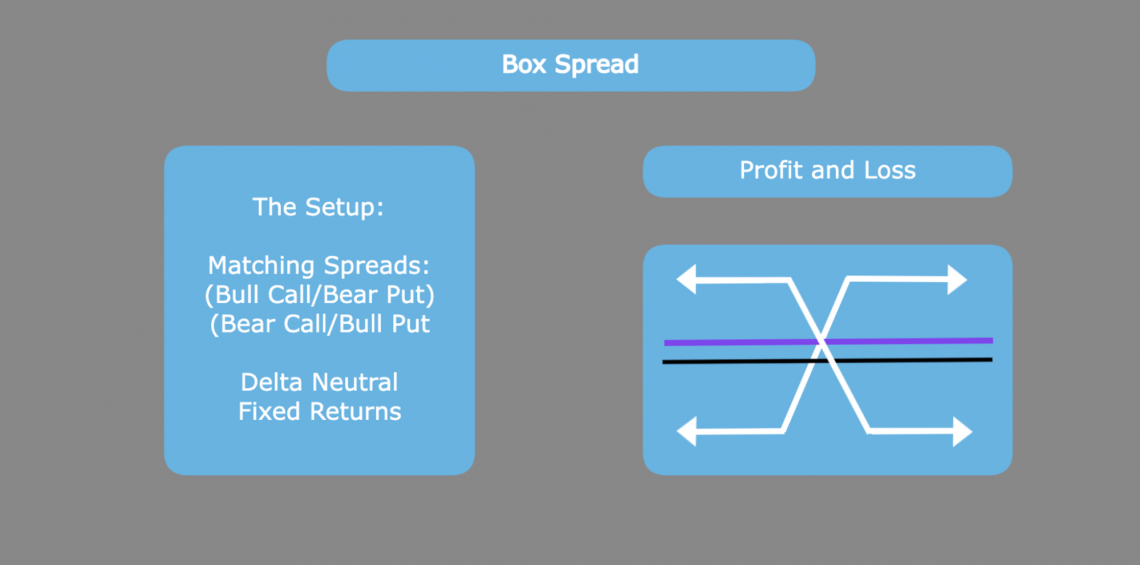Options traders using a box spread are looking to take advantage of options contract pricing arbitrage to generate a fixed return at expiration. This is because combining the four legs creates a delta-neutral strategy that profits the same, regardless of price movement.

Traders can create two types of box spreads, each profiting on different sides of inefficient options pricing. These include:

1. A long box, which looks for underpriced options contracts
2. A short box, which looks for overpriced options contracts

Each of these uses different pairs of vertical distances. These pairs of vertical spaces determine which legs of the strategy are short and long positions

In a long box spread, the investor opens a bull call spread along with a bear put spread. These two spreads will have their respective legs at the same strikes.

Because both the bull call spread and bear put spread are debit spreads, the investor pays a premium to enter this type of box.

In a short box spread, the investor opens a bear call spread, and a bull put spread. These two spreads will also have their respective legs at the same strikes.

Because the box spread has a known return, it is best compared to other fixed-income investments. Therefore, investors will think of their returns in terms of percentage rates.

The strategy may be advantageous if the borrowing or lending rates are better than those offered by their bank or prime broker. This is because the profit or loss is realized at the end, much like a zero coupon bond

As mentioned before, the strategy as a whole depends on being delta-neutral. This guarantees that the returns are the same regardless of price. A combination of matching vertical spreads achieves this goal.One specific strategy, the long box, uses matching debit spreads, while the other strategy, the short tube, uses matching credit spreads.

Overall, there are four types of vertical call spreads that are used. These include the bull call spread, the bear put spread, the bear call spread, and the bull put spread.

Of these four spreads, the two debit spreads matched are the bull call spread and the bear put spread. It follows that the two credit spreads are the remaining two, the bear call spread and the bull put spread.

What does it mean for spreads to be matching?

Matching spreads mean that they share the same strike prices. So, for example, the opposing bull call and bear put feed would share short contracts at the lower strike price and extended warranties at the higher strike price.

This means that the investor's profit and loss are the same regardless of price.

On its own, the bull call spread strategy is a moderately-bullish bet. In this strategy, the investor opens a long call at a lower strike and extends a short call at a higher strike. This strategy is an example of a vertical debit spread, as the investor pays a premium to enter the space.The combination of these two options legs creates a net positive delta, as the lower-strike long call will always be closer to the money or more in the money than the higher-strike short call.

The strategy itself has both a maximum profit and loss. The yield is maximized because the short call limits the upside of the long call. This, however, reduces the premium paid to create a bullish strategy.

The maximum loss of a bull call spread strategy is the total premium paid to enter the spread. Conversely, the maximum profit is the strike difference multiplied by 100 minus the total compensation paid to enter the position.

Therefore, the investor's goal is for the price to increase towards the short leg, where the profit is eventually capped.

On its own, the bear put spread strategy is a moderately-bearish bet. In this strategy, the investor opens a long put at a higher strike and extends a short put at a lower strike. This strategy is an example of a vertical debit spread, as the investor pays a premium to enter the space.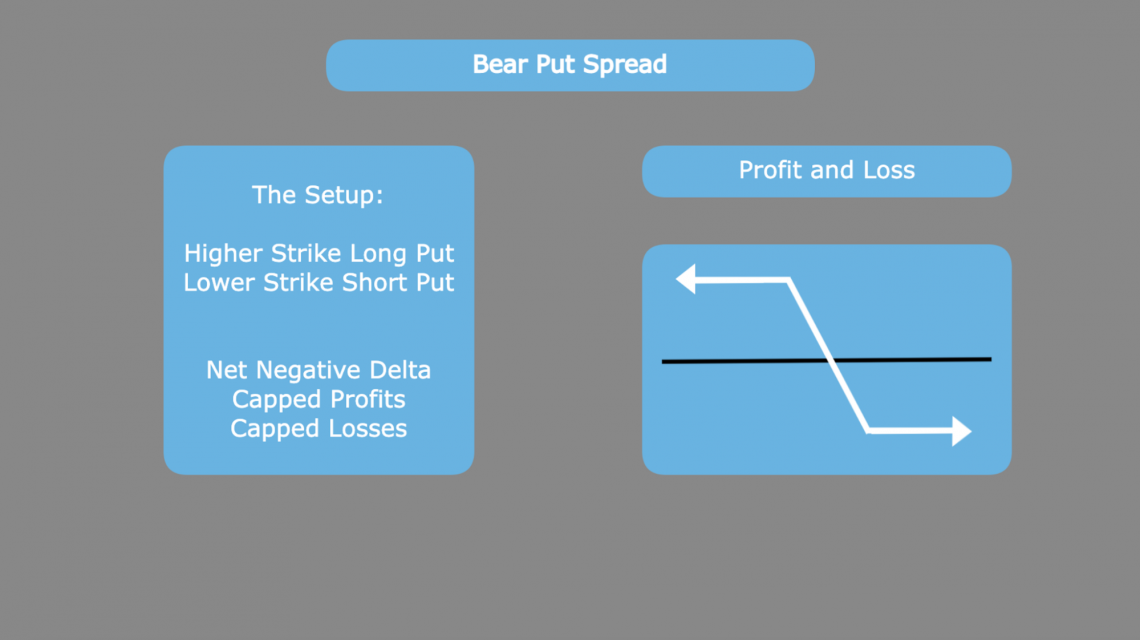The combination of these two options legs creates a net-negative delta, as the higher-strike long put will always be closer to the money or more in the money than the lower-strike short put.

The strategy itself has both a maximum profit and loss. The yield is maximized because the short put limits the upside of the long put. This, however, reduces the premium paid to create a bullish strategy.

The maximum loss of a bear put spread strategy is the total premium paid to enter the spread. Conversely, the maximum profit is the strike difference multiplied by 100 minus the total compensation paid to enter the position.

Therefore, the investor's goal is for the price to decline towards the short leg, where the profit is eventually capped.

On its own, the bear call spread strategy is a moderately-bearish bet. In this strategy, the investor opens a long call at a lower strike and extends a short call at a higher strike.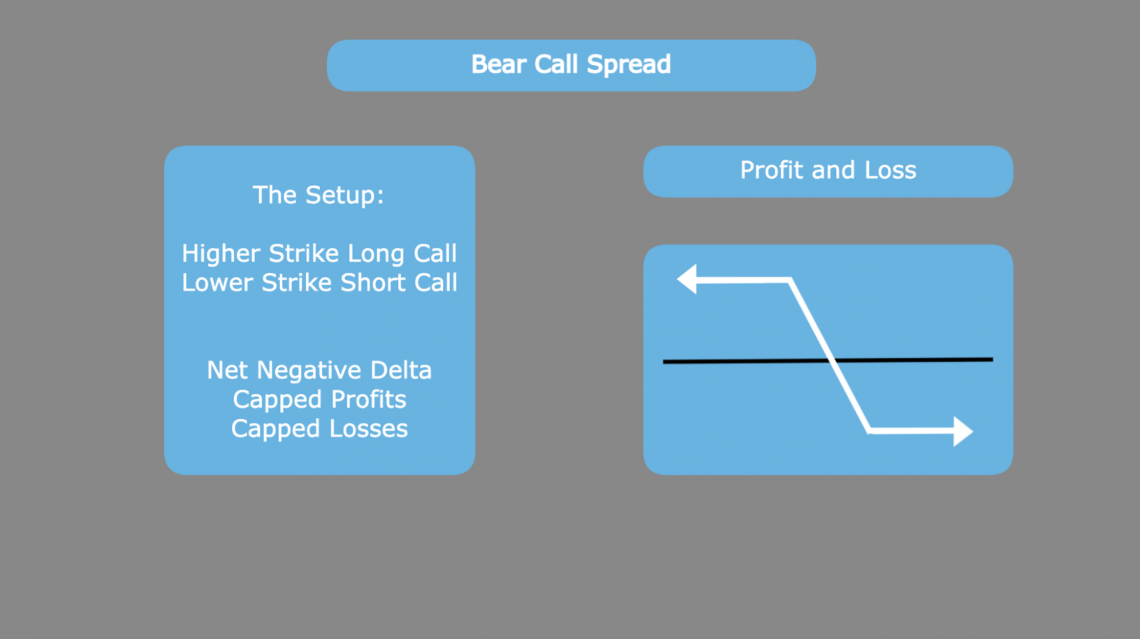This strategy is an example of vertical credit spread, meaning that the investor hopes to retain the premium collected from selling the space.

The combination of these two options legs creates a net-negative delta, as the higher-strike short call will always be farther from the money or less in the money than the lower-strike long call.

The strategy itself has both a maximum profit and loss. The yield is maximized because the premium is initially collected. This total premium is also reduced by the long call, which is opened to hedge the risk that the short position creates.

The maximum profit of a bear call spread strategy is the total premium collected from selling the spread as a whole. Conversely, the maximum loss is the strike difference multiplied by 100 minus the unlimited premium collected to enter the position.

Therefore, the investor's goal is for the price to remain below the short leg, where the profit remains maximized.

On its own, the bull put spread strategy is a moderately-bullish bet. In this strategy, the investor opens a long put at a lower strike and extends a short put at a higher strike.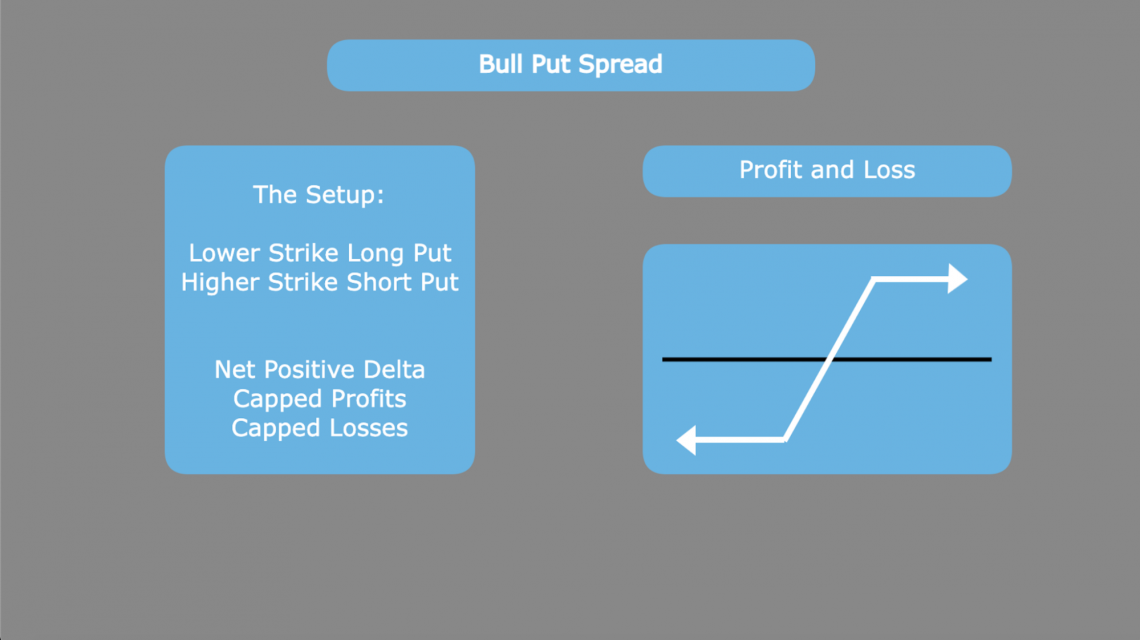This strategy is an example of a vertical credit spread, meaning that the investor hopes to retain the premium collected from selling the space.

The combination of these two option legs creates a net-positive delta, as the lower-strike long put will always be farther from the money or less in the money than the higher-strike short put.

The strategy itself has both a maximum profit and loss. The yield is maximized because the premium is initially collected. This total premium is also reduced by the long put, which is opened to hedge the risk that the short position creates.

The maximum profit of a bull put spread strategy is the total premium collected from selling the spread as a whole. Conversely, the maximum loss is the strike difference multiplied by 100 minus the unlimited premium collected to enter the position.

Therefore, the investor's goal is for the price to remain above the short leg, where the profit remains maximized.

The long box spread is created by matching the bull call, and bear put debit spreads. At expiration, the value of these two spreads will collectively be the difference in strike prices multiplied by 100.

When looking for a box spread, the price of the two debit spreads should be sufficiently below the revenue earned from the strike price differential.

Let's assume that stock XYZ is currently trading for $100 on the open market, and the prices reflect the call and put options for the 95 and 105 strikes expiring in 30 days.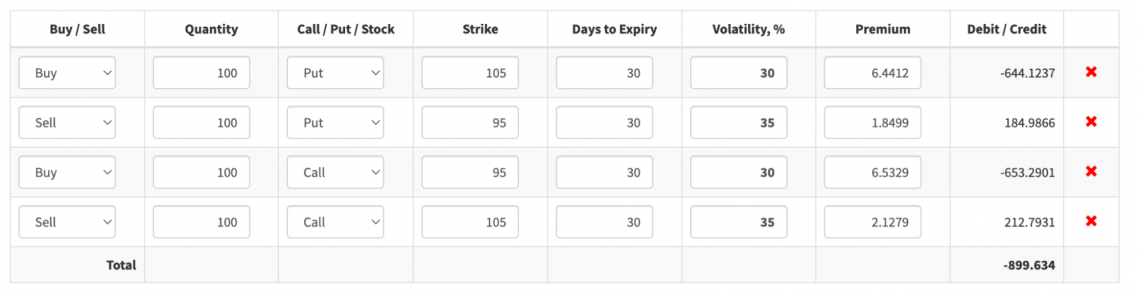Looking at these options, we can split the box into the bear put spread by looking at the two put options and the bull call spread by looking at the two call options It turns out that, in this example, differences in options pricing implied volatility had created a profitable arbitrage opportunity that some investors may capitalize on. If we look at the profit and loss graph, we see that the profit is consistent regardless of price at expiration.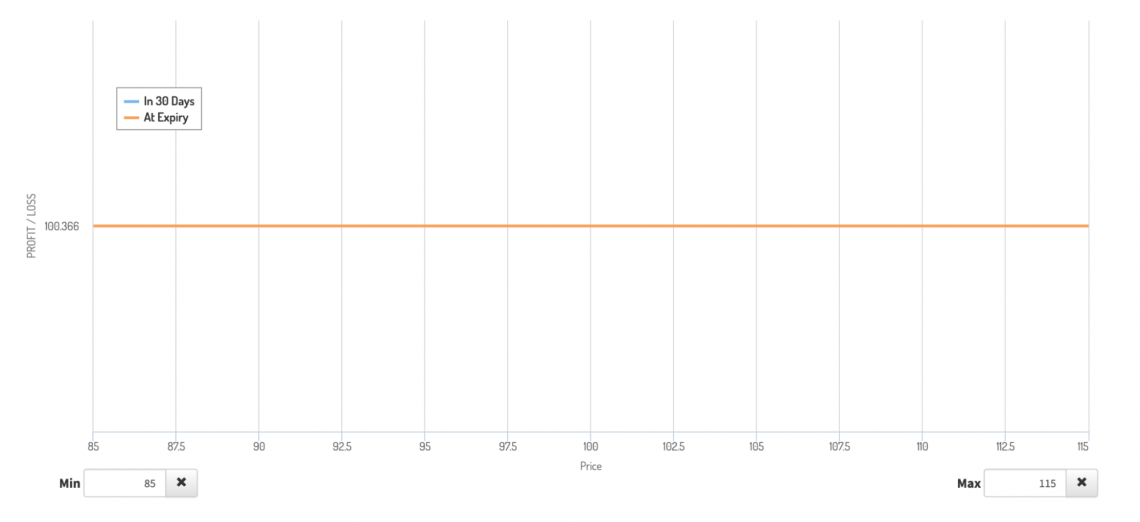Why is this the case? The spread will be worth$1000 at all price points at expiration. This is the difference between the strikes multiplied by 100. The investor is left with $100.36 in profit after subtracting the original premium for all four legs. Profit = (Strike Difference x 100) - Contract Price At price points of 95 and below, the bear put spread profit is maximized, and the bull call spread expires worthless. At price points above 105, the bull call spread profit is maximized, and the bear put expires worthless. At any point in the middle of the two strikes, there is a perfect inverse correlation between the profitability of the two spreads. As one feed gains a dollar of value, the other loses a dollar of value. Let's look at the individual vertical spread profits and losses and see how this plays out.At the$95 expiration, for example, the bear put is worth $1000. Subtracting the original contract price leaves the investor with a profit of about$540.86.At this same price point, the bull call expires worthless. Considering the loss of $440.50 from the bull call, the investor has a final profit of$100.36.

This plays out quite similarly at other expiration prices. For example, at the $105 expiration, the bear put expires worthless, resulting in a loss of around$459.14.

Because the bull call is at the upper strike, its profit is maximized at $560.50. However, the investor once again realizes the exact yield of$100.36.

The short box is the reverse long and short positions seen in the long box. The matching credit spreads are the opposite of those in the long box.

Collecting the premiums associated with the bear call spread and bull put space, the investor is liable for the difference between the two strikes, multiplied by 100 at expiration.

If an investor is looking to earn a profit on the position, the premium earned should be above the liability created by the box. However, if an investor uses this strategy to get a low-cost loan, the bonus must be slightly lower than the liability.

The thinking behind the short position being a loan is that there is a single future payment for which the investor is liable; in return, they get a premium today. This premium can be used as cash, similarly to a loan.

If we take the same options as earlier and switch which calls and puts are sold, we get the short box equivalent of the position.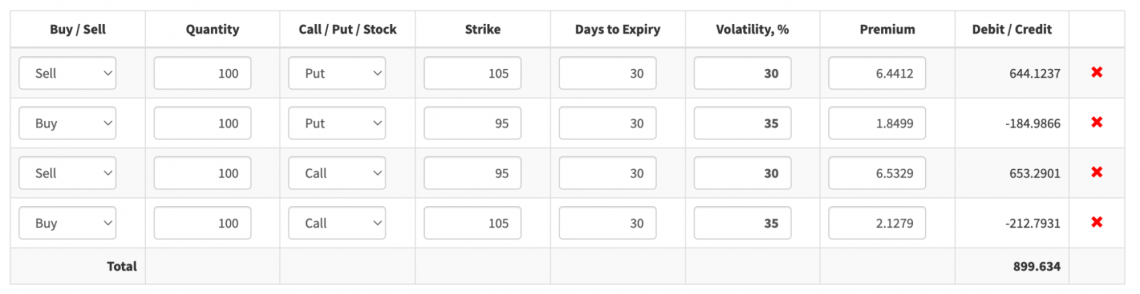Looking at the profit and loss graph, we can see that the $100.36 of profit locked in, regardless of price, is paid for by the person on the opposite side of the contract. So, in this case, the short box holder is guaranteed a$100.36 loss!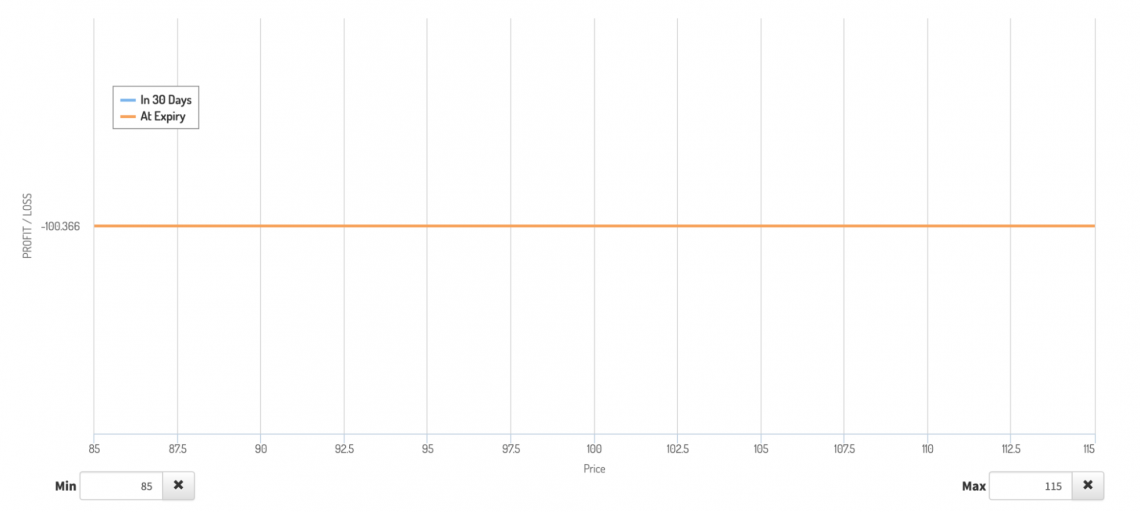Given that the loss in this example is greater than 10% of the premium for just one month of extra cash flexibility, someone is unlikely to open this position to create a synthetic loan.

A far more attractive loan possibility would be one where the period is much more extended, and the premium is closer to the total liability of the box.

We can see this short box as another way to lock in profit if the buy side of the options market is more overpriced. Switching which contracts have greater implied volatility, we get the following short box: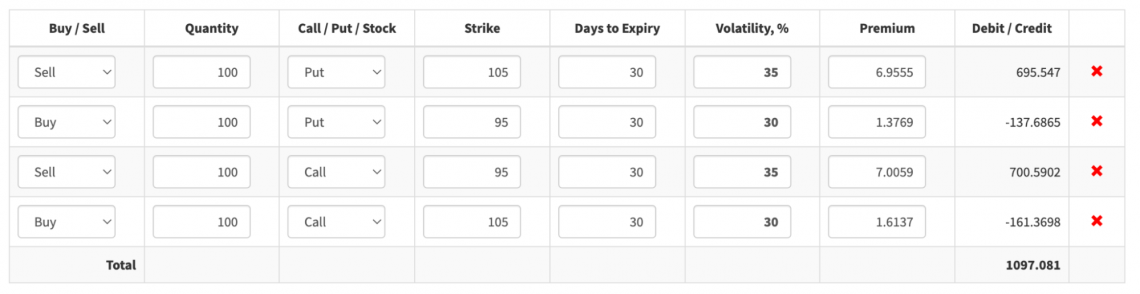Seeing as the investor collects $1097.08 in premium, to begin with, the investor will be guaranteed a profit, as the difference between strikes is only 10. We can easily understand the short box return formula by looking at the profit and loss.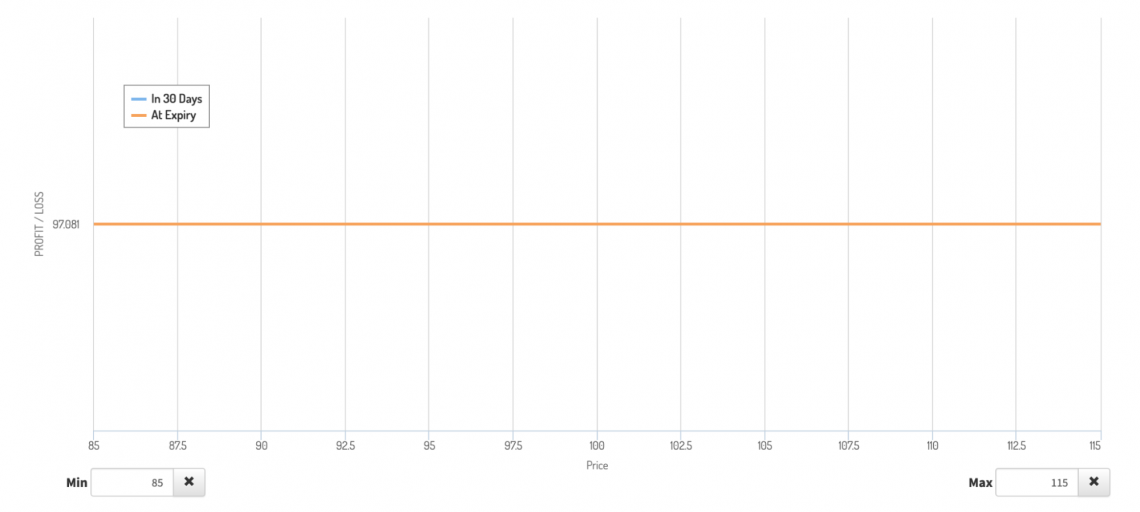Profit = Contract Price - (Strike Difference x 100) In this case, the$1097.08 in premium collected is partially offset by the $1000 in liability, leaving the investor with$97.08 after expiration.

## Final Considerations

While the critical points regarding box spreads can be summarized briefly, investors looking to use this strategy must be aware of other risks the system includes.

First, the key points:

• Box spreads are delta-neutral strategies; investors do not need a directional conviction on the underlying asset.
• The profit or loss on the system is consistent, making them comparable to fixed-income investments.
• This strategy involves four legs that can be broken into two distinct vertical spreads.

Beyond these essential points, a few other aspects can affect the profitability of a box spread succeeding. These aspects must be taken into account before entering a position.

The most obvious of these is a commission for trading options contracts. The profitability is immediately lowered if an investor's broker charges a commission.

While this is the case with all options, it is essential in this situation. More significant price shifts cannot generate price returns that outweigh extra costs.

Furthermore, since this is an arbitrage strategy, the profit margins are not typically lucrative. Commissioning four legs can sharply cut profits more than simple options strategies.

In addition, because of the short legs of the strategy, there is always the chance of early assignment. This is more likely when there is a dividend before expiration.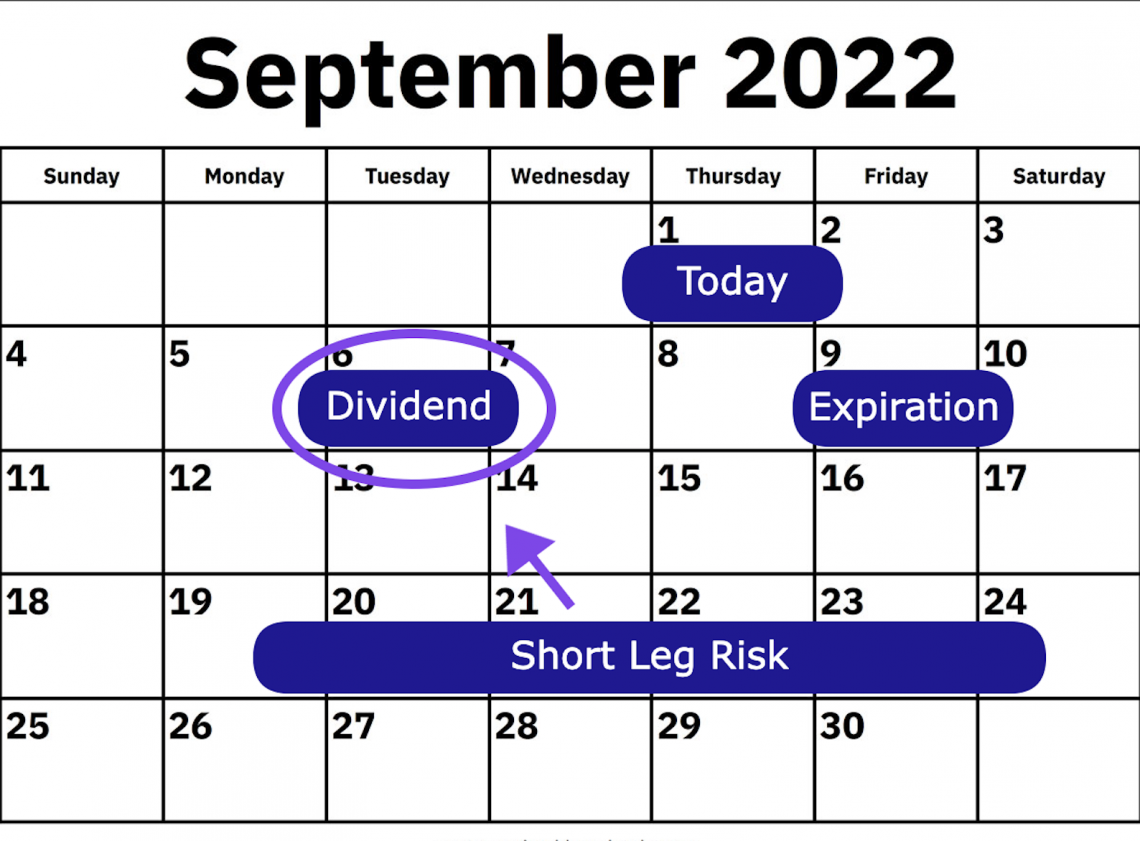Call option holders may exercise if they think the dividend payment will be greater than the remaining time value in the contract. Likewise, put option holders may decide to exercise if they feel the time value exceeds the dividend.

Depending on the account specifications, an early assignment can be pretty crucial. If there is no sufficient balance in the account, liquidation may occur. This is a genuine and extreme risk. It has caused some traders to lose thousands of percent on their positions.

Finally, this strategy is susceptible to interest-rate risk as a fixed-income investment. If interest rates are changed, the fixed income from the process may seem more or less attractive.

To better compare their box spread position to other fixed-income investments, investors can turn their nominal return into an annual percentage. It is pretty simple to do so using Excel. Feel free to check out our Excel course below!### Everything You Need To Master Excel Modeling

To Help You Thrive in the Most Prestigious Jobs on Wall Street.

Researched and authored by Jacob Rounds | LinkedIn